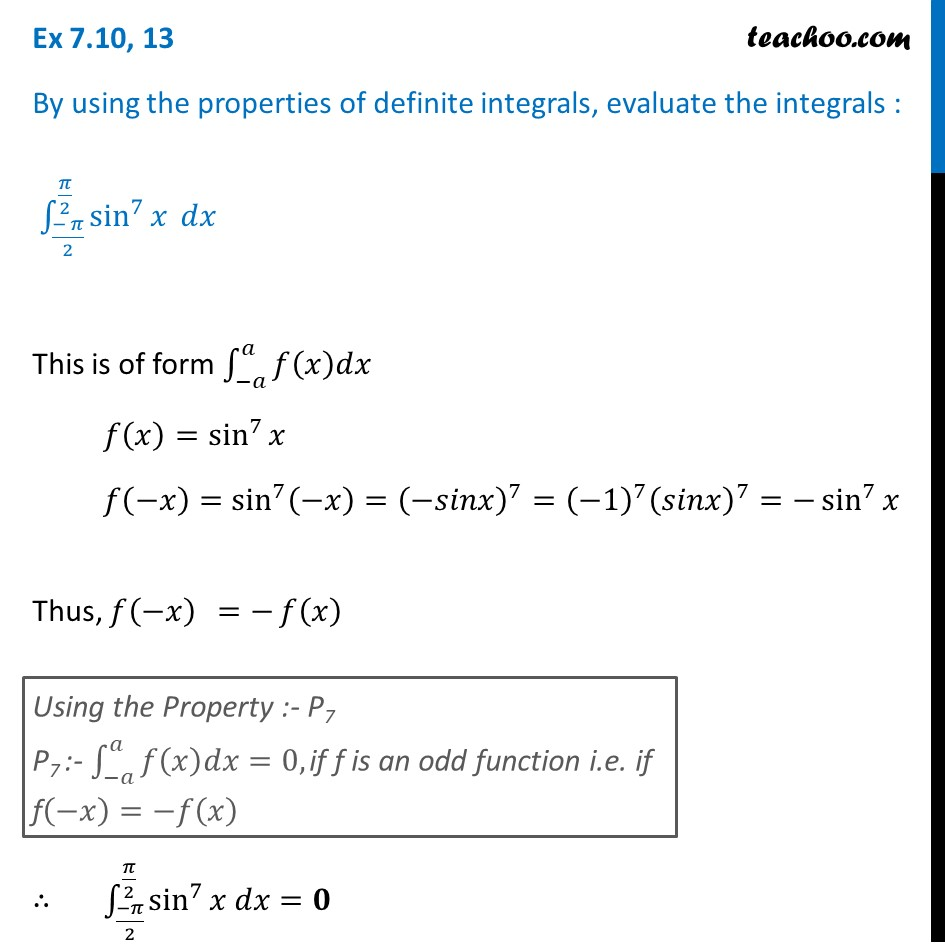Definite Integration by properties - P7

Chapter 7 Class 12 Integrals
Concept wiseLearn in your speed, with individual attention - Teachoo Maths 1-on-1 Class

### Transcript

Ex 7.10, 13 By using the properties of definite integrals, evaluate the integrals : ∫_((− 𝜋)/2)^(𝜋/2)▒sin^7⁡𝑥 𝑑𝑥 This is of form ∫_(−𝑎)^𝑎▒𝑓(𝑥)𝑑𝑥 𝑓(𝑥)=sin^7⁡𝑥 𝑓(−𝑥)=sin^7⁡(−𝑥)=(−𝑠𝑖𝑛𝑥)^7=(−1)^7 (𝑠𝑖𝑛𝑥)^7=−sin^7⁡𝑥 Thus, 𝑓(−𝑥) =−𝑓⁡(𝑥) ∴ ∫_((−𝜋)/2)^(𝜋/2)▒sin^7⁡〖𝑥 𝑑𝑥=𝟎〗